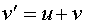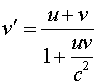Consider two objects. The first object moves with velocity v relative to the second object, while the second object moves with velocity u with respect to an observer. In Newtonian physics the observer would say that the velocity of the first object is the sum of the two velocities.However, this formula would allow the observed velocity to exceed the speed of light, so it cannot be right. When relativistic effects are included, the correct expression is instead found to bewhere we assume u and v are along the same direction. One can check that this resultant velocity never exceeds the speed of light, by working it out for the extreme case that u (or v) equals the speed of light. In that case, v' will be the speed of light. This is consistent with the postulate that the speed of light is the same in all reference frames.

Velocity addition problems can be stated in many ways, often with subtraction of velocities being required rather than addition. A good strategy for approaching these problems is to always write the Newtonian expression first. Then use the relativistic expression above, assigning u and v to whatever velocities are on the right hand side of the expression. If the Newtonian expression has a negative velocity, one must remember to make the velocity in the denominator negative as well.

Relativity index      examples        Lecture index